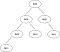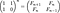Golden Ratio: two quantities are in the golden ratio if their ratio is the same as the ratio of their sum to the larger of the two quantities

# Fibonacci Swift playground

Suppose you are standing in front of a staircase which has `n` stairs. You are allowed to go up either one or two stairs at a time. What will be the total number of distinct ways you can reach to the top?

If we knew the number of ways to get to the both `n-1` and `n-2` , then the total ways to get to the point `n` would be just sum these two values. This is actually same as calculating the `n`th number of the fibonacci series. Here I tried to present different approaches to fibonacci problem which includes algorithm comparison, and discussion around time and space complexity.

## Recursive approach

`func fib(_ n: Int) -> Int {    guard n > 1 else { return n }    return fib(n-1) + fib(n-2)}`

Time Complexity: O(2^n)
Space Complexity: O(2^n)

The problem with this solution is we keep recomputing the same subproblems over and over again. In other words, our algorithm does a lot of repeated work. This algorithm grows exponentially, it takes a lot of effort especially when `n` is large.Recursion tree for fib(4)

## Iterative approach

`func fib(_ n: Int) -> Int {    var fibs: [Int] = [1, 1]    (2...n).forEach { i in         fibs.append(fibs[i - 1] + fibs[i - 2])     }    return fibs.last!}`

Time Complexity: O(n)
Space Complexity: O(n)

With this little change to the algorithm, we made a huge optimization. As an example for `n = 45` it takes 90 steps (whereas over a billion with our recursive approach), roughly 10 million times faster.

## Iterative approach — Optimized

`func fib(_ n: Int) -> Int {    var a = 1    var b = 1    guard n > 1 else { return a }        (2...n).forEach { _ in         (a, b) = (a + b, a)     }    return a}`

Time Complexity: O(n)
Space Complexity: O(1)

Inside the for loop, we are assigning (beauty of the tuples) the new values to both `a` and `b`. If you noticed the equation `(a, b) = (a + b, a)` is the exact definition of the golden ratio.

## Power of Matrix approach

In order to have the `n`th Fibonacci number, the matrix M has to be multiplied to itself `n-1` times, and form the `n-1`th power:Fibonacci closed-form expression, Binet’s formula

Let’s say we have an awesome method `multiply` that multiplies two matrixes and returns a resultant matrix, the algorithm will be as simple as this:

`func fib(_ n: Int) -> Int {    guard n > 2 else { return n }        let M = [[1, 1], [1, 0]]    var result: [[Int]] = M    (1..<n).forEach { _ in result = multiply(result, M) }    return result}`

Time Complexity: O(n)
Space Complexity: O(1)

## Power of Matrix approach — Optimized

As you see this squaring approach uses many fewer multiplications than the primitive approach (three vs. eight, in other word reduced logarithmic). The same idea works for matrices:

`func fib(_ n: Int) -> Int {    var M = [[1, 1], [1, 0]]    guard n > 2 else { return n }    power(&M, n)    return M}func power(_ matrix: inout [[Int]], _ n: Int) {    guard n > 1 else { return }    power(&matrix, n/2)    matrix = multiply(matrix, matrix)    if n % 2 != 0 {        let M = [[1, 1], [1, 0]]        matrix = multiply(matrix, M)    }}`

Time Complexity: O(log n)
Space Complexity: O(log n) , because of recursion stack

In the code snippet above the `power` function tries to compute the `n`th power of `M` by squaring the`(n/2)`th power. However if n is odd, rounding down `n/2` and squaring that power of `M` results in the `(n-1)`th power, which multiplying one more factor of `M` will makes up that single factor. This is for sure the fastest and most optimized algorithm we can use to get the `n`th fibonacci number.

You might be asking about `inout` keyword or `&` sign, these are not really playing any significant role in our algorithm. They just let us pass the matrix reference through the recursion stack for the performance purposes. If you are interested to learn more, check out Value Types vs. Reference Types in Swift.

If you have any suggestions, please let me know, and feel free to leave your comment. You can find all the code for this post here.

# Further Study:

This post is to Alex Smirnoff who is always motivating

## The Startup

Get smarter at building your thing. Join The Startup’s +724K followers.

## The Startup

Get smarter at building your thing. Follow to join The Startup’s +8 million monthly readers & +724K followers.

Written by

## Masih Tabrizi

iOS Developer, computer science freak## The Startup

Get smarter at building your thing. Follow to join The Startup’s +8 million monthly readers & +724K followers.

## More From Medium

Medium is an open platform where 170 million readers come to find insightful and dynamic thinking. Here, expert and undiscovered voices alike dive into the heart of any topic and bring new ideas to the surface. Learn more

Follow the writers, publications, and topics that matter to you, and you’ll see them on your homepage and in your inbox. Explore

If you have a story to tell, knowledge to share, or a perspective to offer — welcome home. It’s easy and free to post your thinking on any topic. Write on Medium